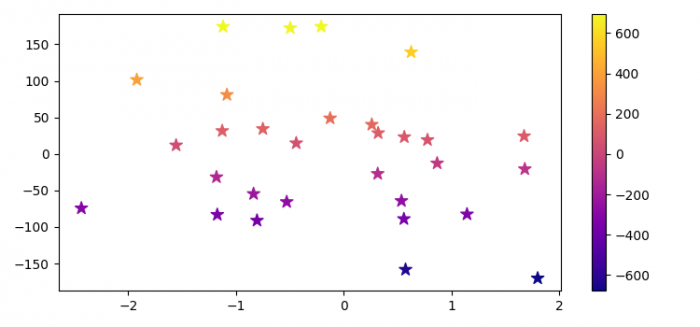# How to plot a multivariate function in Python Matplotlib?

To plot a multivariate function in Python, we can take the following steps −

## Steps

• Set the figure size and adjust the padding between and around the subplots.

• Create random x, y and z data points using numpy.

• Create a figure and a set of subplots.

• Create a scatter plot with x, y and z data points.

• Create a colorbar for a ScalarMappable instance, s.

• To display the figure, use show() method.

## Example

import numpy as np
import matplotlib.pyplot as plt

plt.rcParams["figure.figsize"] = [7.50, 3.50]
plt.rcParams["figure.autolayout"] = True

def func(x, y):
return 3 * x + 4 * y - 2 + np.random.randn(30)

x, y = np.random.randn(2, 30)
y *= 100
z = func(x, y)

fig, ax = plt.subplots()

s = ax.scatter(x, y, c=z, s=100, marker='*', cmap='plasma')

fig.colorbar(s)

plt.show()

## Output

It will produce the following output −